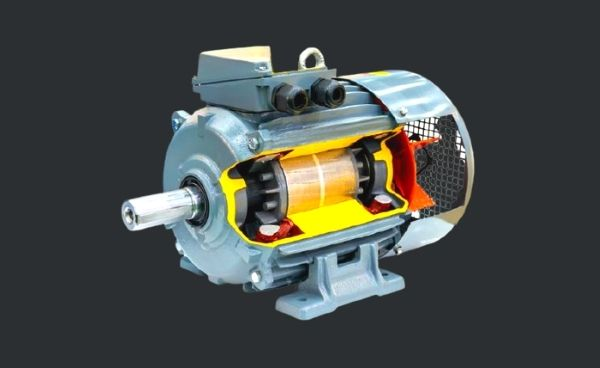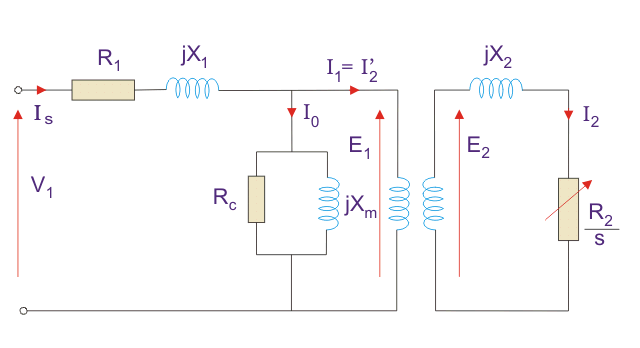# why does induction motor draws a high current at startingThe induction motor draws a high starting current as compared to the running condition. The starting current of the induction motor is about 5 or 6 times the motor full load current. An induction motor of rating 11 KW,22 Amps,440 volts takes a high starting current of about 132 Amps. The current reduces as the motor accelerates towards its base speed or synchronous speed.

## Why High Starting Currents in induction motor

### Reason 1: Due to the inductive characteristics

The induction motor may be regarded as a Transformer with a primary coil and a secondary coil loaded with a load that changes its impedance.figure 1: Equivalent circuit of induction motor

The secondary coil is normally a one-turn coil.

The impedance of the load at the rotor is made up of low resistance R and low inductance L hence,

```Z=(R+jwL)

where,
w - Frequency
R - Resistance
L - inductance
Z - Impedance```

where W is the frequency that changes with the rotation of the rotor till it becomes Zero when the rotor reaches synchronous speed.

When the rotor of an induction motor is stationary the current in the shorted conductive loops of the rotor is very high as the resistance and the inductance is low while the effective frequency is that of the supply frequency.

This high current in the rotor will create its own magnetic field which opposes the main stator magnetic field, this weakens the stator magnetic field so the back EMF in the stator will drop and the supply voltage will be much higher than the stator back EMF and so the supply current increases to a high value. Those are the starting conditions.

As far as impedance goes the rotor impedance is defined by Z=(R+jwL) were w, the frequency and this is high at starting and as the rotor starts turning the frequency in the rotor will decrease till it is zero at synchronous speed. So, in fact, the impedance in the rotor is decreasing as the rotor speed up.

### Reason 2 : Due to starting slip of the motor

The voltage induced in the rotor depends on the relative speed of the synchronous speed of the rotating magnetic field and the speed of the rotor.

At the start, the rotor is at a standstill so its speed is equal to zero. At starting, the difference between the speed of the synchronous speed of the rotating magnetic field and the speed of the rotor is maximum.

The difference between the synchronous speed and the rotor speed is called of the motor.

```The slip of the motor can be expressed as;

S = (Ns- Nr)/Ns *100   ---------(1)

Where,
S = Slip
Ns= Synchronous speed of the motor= 120 f/P
Nr = Rotor speed```

As the speed of the rotor is zero at the start, the rotor conductor will cut the maximum flux and the maximum voltage will be induced in the rotor.

As the motor starts accelerating the speed of the rotor will align in the direction of synchronous speed of the motor and the slip will get reduced.

The voltage induced in the rotor conductor can be expressed as;

```Er = S* Es ------------(2)

Where,
Er = Rotor Voltages
S = Slip
Es = Stator Voltage```

At start, the slip of the motor is equal to unity and the induced rotor voltage is equal to the stator voltage. The rotor-induced voltage goes on decreasing as the motor accelerates towards its base speed.

```Er = Es
When Nr =0 & slip=1```

It is clear that the induced rotor is maximum at the starting of the motor.

Autotransformer starter and VFD drives used to limit the starting current of induction motor.

### Disadvantages of High Starting Currents in Induction Motor

1. High inrush currents

High inrush currents drawn by induction motor during starting can result in large dip in connected bus voltages.

This dip in bus voltages can impact the performance of other motors operating on the bus. Voltage dips during starting of large motors can trip some of the motors operating on the same bus.

Care should be taken to limit the inrush currents during starting of the motor by employing proper starting methods

2. Increase in the temperature of the machine

For large motors life of the machine depends on the number of startings. High inrush currents can cause an increase in the temperature of the machine, damages the insulation, and can reduce the life of the machine